# Texas Go Math Grade 8 Lesson 4.5 Answer Key Solving Systems of Linear Equations by Graphing

Refer to our Texas Go Math Grade 8 Answer Key Pdf to score good marks in the exams. Test yourself by practicing the problems from Texas Go Math Grade 8 Lesson 4.5 Answer Key Solving Systems of Linear Equations by Graphing.

## Texas Go Math Grade 8 Lesson 4.5 Answer Key Solving Systems of Linear Equations by Graphing

Explore Activity
Investigating Systems of Equations

You have learned several ways to graph a linear equation in slope-intercept form. For example, you can use the slope and y-intercept or you can find two points that satisfy the equation and connect them with a line.

A. Graph the pair of equations together: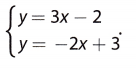B. Explain how to tell whether (2, -1) is a solution of the equation y = 3x – 2 without using the graph.

C. Explain how to tell whether (2, -1) is a solution of the equation y = -2x + 3 without using the graph.

D. Explain how to use the graph to tell whether the ordered pair (2, -1) is a solution of either equation.

E. Find the point of intersection of the two lines. Check by substitution to determine if it is a solution to both equations.Reflect

Question 1.
A system of linear equations has infinitely many solutions. Does that mean any ordered pair in the coordinate plane is a solution?
When a system of linear equations has infinitely many solutions, it means that graphing those equations will give us the same line. Therefore, the solutions of this system would be all the ordered pairs that lie on this line. Having infinitely many solutions does not mean that any ordered pair ¡n the coordinate plane is a solution.

Can you show algebraically that both equations in part B represent the same line? If so, explain how.
y = 3x – 3
Distribute 3 to the terms within the parentheses.
y = 3(x – 1) = 3x – 3
Both equations in part B represent the same line.

y = 3(x – 1) = 3x – 3

Solve each system by graphing. Check by substitution.

Question 3.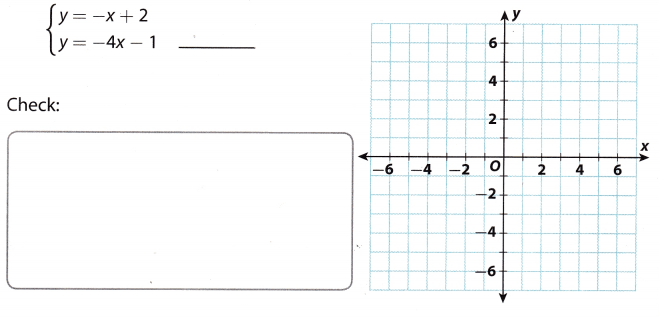y = -x + 2
y = -4x – 1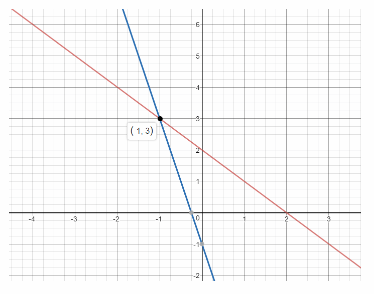Graph each of the equations.
Solution: (-1, 3) The solution of the linear systems is the intersection of the two equations

3 = -(-1) + 2 Check by substituting in the original equations
3 = 1 + 2
3 = 3
y = -4x – 1
3 = -4(-1) – 1
3 = 4 – 1
3 = 3

Question 4.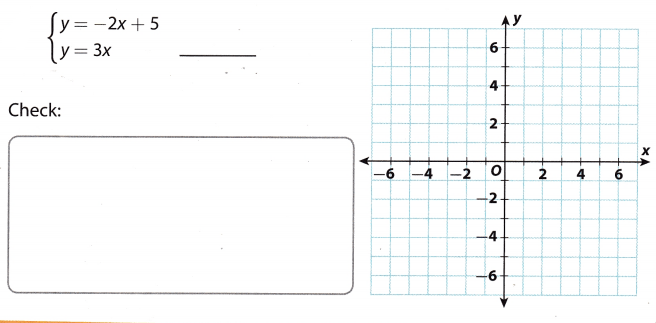Start by graphing each equation.
Find the point of intersection of the two lines.
It appears to be (1, 3). Check by substitution to determine if it is a solution to both equations.
y = -2x + 5
3 = -2(1) + 5
3 = -2 + 5
3 = 3
y = 3x
3 = 3(1)
3 = 3
The solution of the system is (1, 3).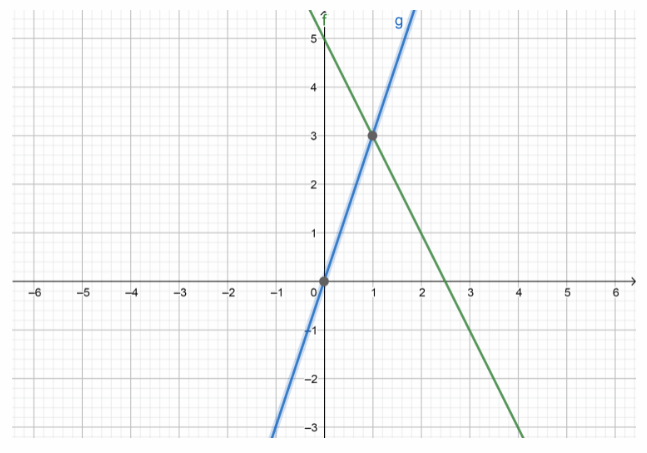The solution of the system (1, 3)

Reflect

Question 5.
Conjecture Why do you think the graph is limited to the first quadrant?
The solution is restricted to the first quadrant as the number of hot dogs and the number of drinks cannot be negative.

During school vacation, Marquis wants to go bowling and play laser tag. He wants to play 6 total games but needs to figure out how many of each he can play if he spends exactly $20. Each game of bowling is$2 and each game of laser tag is $4. a. Let x represent the number of games Marquis bowls and let y represent the number of games of laser tag Marquis plays. Write a system of equations that describes the situation. Then write the equations in slope-intercept form. Answer: x + y = 6 Let x be the number of bowling games and y be the number of laser tag games 2x + 4y = 20 x + y – x = -x + 6 Rewrite in slope-intercept form y = -x + 6 2x + 4y – 2x = -2x + 20 4y = -2x + 20 y = –$$\frac{2}{4}$$x + $$\frac{20}{4}$$ y = –$$\frac{1}{2}$$x + 5 b. Graph the solutions of both equations. Answer:Graph the equations on the same coordinate plane y = -x + 6 y = –$$\frac{1}{2}$$x + 5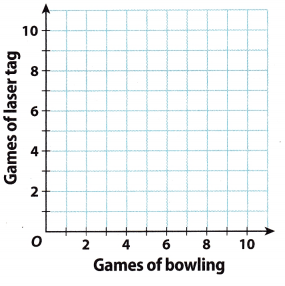c. How many games of bowling and how many games of laser tag will Marquis play? Answer: Marquis should play 2 games of bowling and 4 games of laser tag Solution Texas Go Math Grade 8 Lesson 4.5 Guided Practice Answer Key Question 1.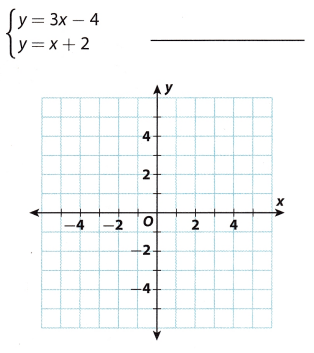Answer: y = 3x – 4 y = x + 2 We are given a system of equations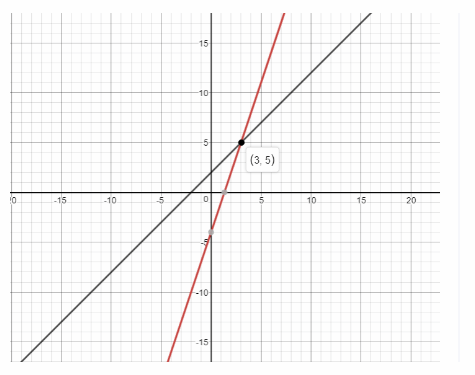Graph the equations on the same coordinate plane (3, 5) is the solution of the system of equations. The solution of the linear system of equations is the intersection point of the two equations. y = 3x – 4 Check by substitution to determine if it is a solution to both equations. 5 = 3.3 – 4 5 = 9 – 4 5 = 5 True y = 3 + 2 5 = 5 True (3, 5) is the solution of the system of linear equations. Go Math 8th Grade Answer Key Lesson 4.5 Question 2.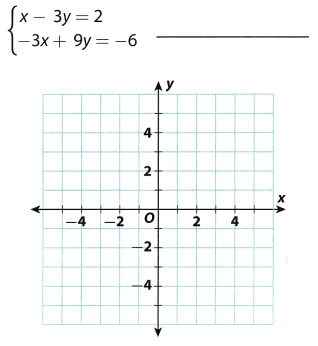Answer: x – 3y = 2 Given -3x + 9y = -6 x – 3y – x = -x + 2 Rewrite in slope-intercept form -3y = -x + 2 y = $$\frac{1}{3}$$x – $$\frac{2}{3}$$ -3x + 9y + 3x = 3x – 6 9y = 3x – 6 y = $$\frac{3}{9}$$x – $$\frac{6}{9}$$ y = $$\frac{1}{3}$$x – $$\frac{2}{3}$$Graph the equations on the same coordinate plane Solution: Infinitely many solutions The solution of the linear system of equations is the intersection of the two equations Question 3. Mrs. Morales wrote a test with 15 questions covering spelling and vocabulary. Spelling questions (x) are worth 5 points and vocabulary questions (y) are worth 10 points. The maximum number of points possible on the test is 100. (Example 2) a. Write an equation in slope-intercept form to represent the number of questions on the test. Answer: x + y = 15 The sum of two types of questions is 15 x + y – x = -x + 15 Rewrite in slope-intercept form y = -x + 15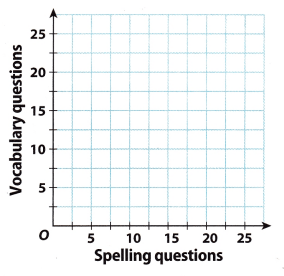b. Write an equation in slope-intercept form to represent the total number of points on the test. Answer: 5x + 10y = 100 The total number of points on the test is 100 5x + 10y – 5x = -5x + 100 Rewrite in slope-intercept form 10y = -5x + 100 y = –$$\frac{5}{10}$$x + $$\frac{100}{10}$$ y = –$$\frac{1}{2}$$x + 10 c. Graph the solutions of both equations. Answer: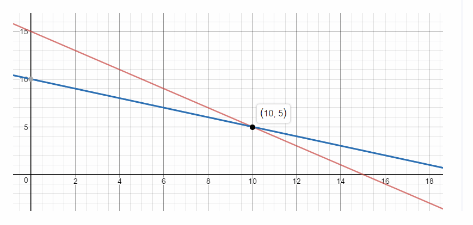Graph the equations on the same coordinate plane d. Use your graph to tell how many of each question type are on the test. Answer: Solution: (10, 5) There should be 10 spelling questions and 5 intersections of the two equations. The solution of the linear system of equations is the vocabulary questions. Essential Question Check-In Question 4. When you graph a system of linear equations, why does the intersection of the two lines represent the solution of the system? Answer: To solve a system of linear equations means to find the solutions that satisfy all the equations of that system. When we graph a system of linear equations, the intersection point lies on the line of each equation, which means that satisfies all, the equations. Therefore, it is considered to be the solution for that system. Texas Go Math Grade 8 Lesson 4.5 Independent Practice Answer Key Question 5. Vocabulary A _________ is a set of equations that have the same variables. Answer: A “system of equations” is a set of equations that have the same variables. Go Math Answer Key Grade 8 System of Linear Equations Question 6. Eight friends started a business. They will wear either a baseball cap or a shirt imprinted with their logo while working. They want to spend exactly$36 on the shirts and caps. The shirts cost $6 each and the caps cost$3 each.
a. Write a system of equations to describe the situation. Let x represent the number of shirts and let y represent the number of caps.
_________
x – the number of shirts;
y – the number of caps;
(The sum of all shirts and caps.)x + y = 8
(The total cost of all shirts and caps is 36 dollars.)6x + 3y = 36

b. Graph the system to find the solution. Verify the solution. What does it represent?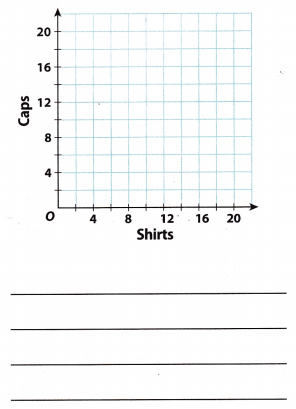Write the equations in slope-intercept form. Then graph.
x + y = 8
y = 8 – x
y = -x + 8 and (Divide both sides by 3.)
6x + 3y = 36
2x + y = 12
y = -2x + 12
Graph the equations y = -x + 8 and y = -2x + 12.
Use the graph to identify the solution of the system of equations.
Apparent solution: (4, 4)
x + y = 8
4 + 4 = 8
8 = 8
and
6(4) + 3(4) = 36
24 + 12 = 36
36 = 36
The point (4, 4) is a solution of both equations.
Eight friends should order 4 shirts and 4 caps.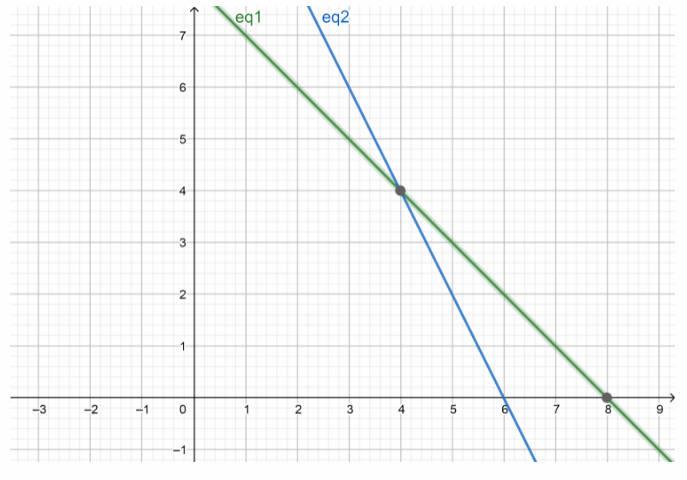The point (4, 4) is a solution of both equations
Eight friends should order 4 shirts and 4 caps

Question 7.
Multistep The table shows the cost for bowling at two bowling alleys.a. Write a system of equations, with one equation describing the cost to bowl at Bowl-o-Rama and the other
describing the cost to bowl at Bowling Pinz. For each equation, let x represent the number of games played and let y represent the total cost.
x – the number of games played;
y – the total cost;
(The total cost to bowl at Bowl-o-Rama)y = 2 + 2.5x
(The total cost to bowl at Bowling Pinz)y = 4 + 2x

b. Graph the system to find the solution. Verify the solution. What does it represent?Graph the equations y = 2 + 2.5x and y = 4 + 2x.
Use the graph to identify the solution of the system of equations.
Apparent solution: (4, 12)
y = 2 + 2.5x
12 = 2 + 2.5(4)
12 = 2 + 10
12 = 12 and
y = 4 + 2x
12 = 4 + 2(4)
12 = 4 + 8
12 = 12
Point (4, 12) is a solution or both equations.
The total cost for four games is 12 dollars.The point (4, 12) is a solution of both equations.
The total cost for four games is 12 dollars.

Graphing Linear Equations Vocabulary Answer Key Question 8.
Multi-Step Jeremy runs 7 miles per week and increases his distance by 1 mile each week. Tony runs 3 miles per week and increases his distance by 2 miles each week. In how many weeks will Jeremy and Tony be running the same distance? What will that distance be?
Jeremy’s distance after x weeks:
y = x + 7
Tony’s distance after x weeks:
y = 2x + 3
To find after how many weeks will Jeremy and Tony be running the same distance and what will the distance be, we can solve the system of those two linear equations:
y = x + 7
y = 2x + 3
We graph each equation on the same coordinate plane and we find the intersection point of those two lines, which is the solution of the system.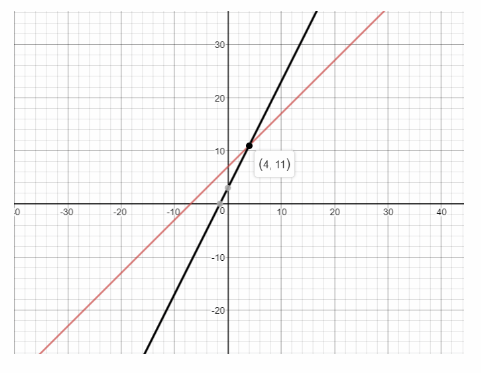The solution of the system of linear equation is (4, 11), which means that after 4 weeks Jeremy and Tony will be running the same distance and that distance would be 11 miles.

After 4 weeks Jeremy and Tony will be running the same distance and that distance would be 11 miles.

Question 9.
Critical Thinking Write a real-world situation that could be represented by the system of equations shown below.Explanation A:
The entry fee for the first gym is $10 and for every hour that you spend there, you pay an extra$. If we denote with x the number of hours that somebody spends at the gym and with y the total cost, we have:
y = 4x + 10
On the other hand, the entry fee of the second gym is $15 and for every hour that you spend there, you pay an extra$3. If we denote with x the number of hours that somebody spends at the gym and with y the total cost, we
have:
y = 3x + 15
To find after how many hours the costs of the gyms will be the same and what will that cost be, we can solve the system of linear equations:
y = 4x + 10
y = 3x + 15

Let y be the total cost of skating and x be the rental cost of the stakes per hour. The constant represents the entry fee to the skating arena. In the first equation, $4 per hour is the rental cost of stakes and$10 is the entry fee. In the second equation, $3 per hr is the rental cost of stakes and$15 is the entry fee.

Texas Go Math Grade 8 Lesson 4.5 H.O.T. Focus On Higher Order Thinking Answer Key

Multistep The table shows two options provided by a high-speed Internet provider.a. In how many months will the total cost of both options be the same? What will that cost be?
x – the number of months;
y – the total cost of one option after x months;
(The total cost for Option 1) after x months y = 50 – 30x
(The total cost for Option 2) after x months y = 40x
Check-in how many months the total cost of both options will be the same.
(Subtract 30x from both sides.)40x = 50 + 30x
(Divide both sides by 10.)10x = 50
(The total cost of both options will be the same in 5 months.)x = 5
(For x = 5)y = 40x
y = 40(5)
(The total cost will be 200 dollars.)y = 200

b. If you plan to cancel your Internet service after 9 months, which is the cheaper option? Explain.
y = 50 + 30(9)
y = 50 + 270
(The total cost for Option 1) after 9 months y = 320 dollars
y = 40x
y = 40(9)
(The total cost for Option 2) after 9 months y = 360 dollars
After 9 months Option 1 is cheaper than Option 2

Texas Go Math Grade 8 Pdf Linear Equations Vocabulary Question 11.
Draw Conclusions How many solutions does the system formed by x – y = 3 and ay – ax + 3a = 0 have for a nonzero number a? Explain.